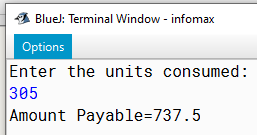# Java program to input number of unit electricity consumed and calculate electricity bill

An electricity company charges their consumers according to the units consumed per month according to the given traffic:

 Units Consumed Charges Up to 100 units ₹ 2 per unit More than 100 units and up to 200 units ₹ 1.80 per unit More than 200 units ₹ 1.50 per unit

In addition to the above, every consumer has to pay ₹ 200 as Service Charge per month. Write a program to input the amount of units consumed and calculate the total charges payable (Bill) by the consumer.

`Solution`

``````import java.util.*;
class Bill
{
public static void main(String arr[])
{
float u,c;
Scanner sc=new Scanner(System.in);
System.out.println("Enter the units consumed:");
u=sc.nextInt();
if (u<=100)
c=2*u;
else if (u<=200)
c=100*2+(u-100)*1.8f;
else
c=100*2+100*1.8f+(u-200)*1.50f;
c=200+c;
System.out.println("Amount Payable="+c);
}
}``````
`Output`